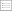Print this page

DISCUSSION PAPER PI-0811

Optimal Pension Asset Allocation Strategy for Defined-contribution Plans with Exponential Utility

Qing-Ping Ma

This paper considers the asset allocation strategies for members of
defined-contribution pension plans with exponential utility when there are three types
of asset, cash, bonds and stocks. The portfolio problem is to maximize the expected
utility of terminal wealth that uses the plan member’s final wage as a numeraire, in
the presence of three risk sources, interest risk, asset risk and wage risk. The use of a
stochastic numeraire makes usual riskless cash assets risky. A closed form solution is
found for the asset allocation problem when a portfolio replicates exactly the wage
process exists, which is the true riskless asset. The optimal portfolio composition is
horizon dependent, while the investments in the three asset classes have constant
wealth-to-wage ratios. The paper discusses the implication of using wage as
numeraire and assuming exponential utility function in portfolio and pension
investment strategy studies.

Keywords : Defined-contribution pension plan; Wage risk; Optimal asset allocation;
Exponential utility; Hamilton-Jacobi-Bellman equation.